# Nearest greater to left

Category: Data Structures
Tags: #datastructures#stack

Write a program to find the nearest greater element or next greater to the left of each element in an array.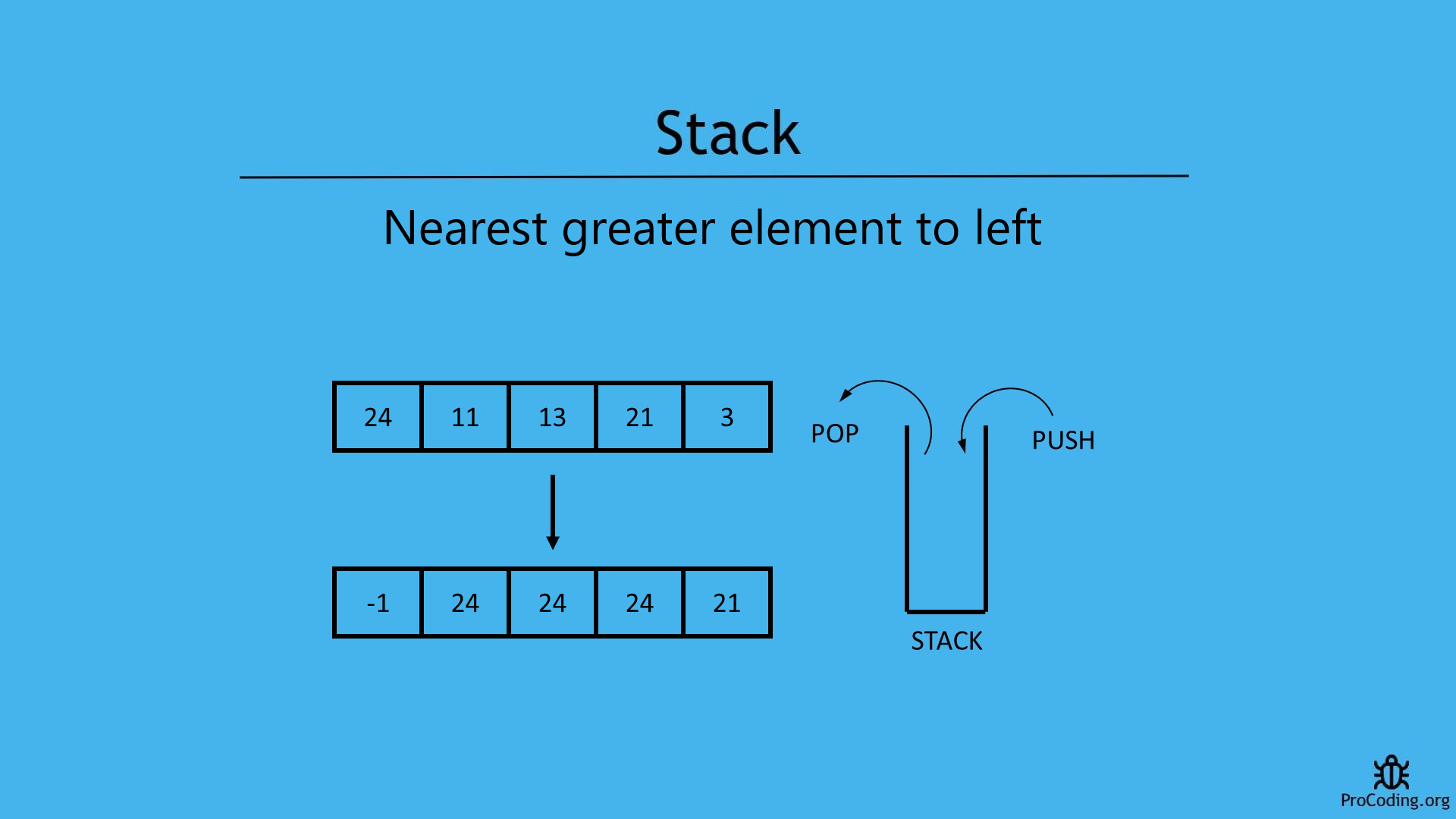### Problem

In case of this problem, an array is given and we have to find first greater element to the left of current element. Similalrly, find the greater elements for each element in the array and if greater element is not available then return a default value based on the problem.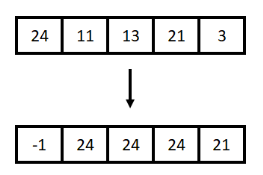Example -

``````Input: [4, 5, 2, 0]
Output: [-1, -1, 5, 2]

Input: [1, 6, 4, 10, 2, 5]
Output: [-1, -1, 6, -1, 10, 10]
``````

Few points to consider:

1. If the array is sorted in increasing order, next greater element will always be -1.
2. For the left most element, left greater element will always be -1.

We will see two ways to solve the problems

## Brute-force approach (using nested loops)

In this approach we will use two loops. Outer loop will iterate over all the array items and inner loop will iterate over all the previous elements of currently pointed by outer loop. Inner loop will check, if any greater element will be found then loop will be terminated and if greater element is not found then `-1` will be added as result.

Python
``````def nearest_greater_to_left(arr):
result = []
for i in range(0, len(arr)):
for j in range(i-1, -1, -1):
if arr[i] < arr[j]:
result.append(arr[j])
break
else:
result.append(-1)

return result

arr = [1, 6, 4, 10, 2, 5]
print(nearest_greater_to_left(arr))
``````
JavaScript
``````function nearestGreaterToLeft(arr) {
let result = [];
for (let i = 0; i < arr.length; i++) {
let flag = false;
for (let j = i - 1; j > -1; j--) {
if (arr[i] < arr[j]) {
result.push(arr[j]);
flag = true;
break;
}
}
if (!flag) {
result.push(-1);
}
}

return result;
}

arr = [1, 6, 4, 10, 2, 5];
console.log(nearestGreaterToLeft(arr));
``````

Output

``````[-1, -1, 6, -1, 10, 10]
``````

Although, we have solved this problem using loops but this is not an optimized solution. Because time complexity of this solution is O(n^2).

## Using stack

Algorithm

``````NearestGreaterToLeft(A)
1. for i = A.length to 0
2.   if stack is empty
4.     stack.push(A[i])
5.   else if not stack is empty
6.     while (stack is not empty AND A[i] > top of stack)
7.       stack.pop()
8.     if stack is empty
10.    else
12.    stack.push(A[i])
13. return reverse of result
``````

In this approach, we are using a stack.

We have to start traversing elements of the given array from the beginning i.e., leftmost element and we will be putting the highest element in the stack and whenever we get another highest element then pop the stack and push the new element. Basically, we will be using a stack to keep values available to the left side and which are the greater ones.

If the stack is empty the greater element to left will be `-1` otherwise top of stack will be the highest element. At the end we have return the result array.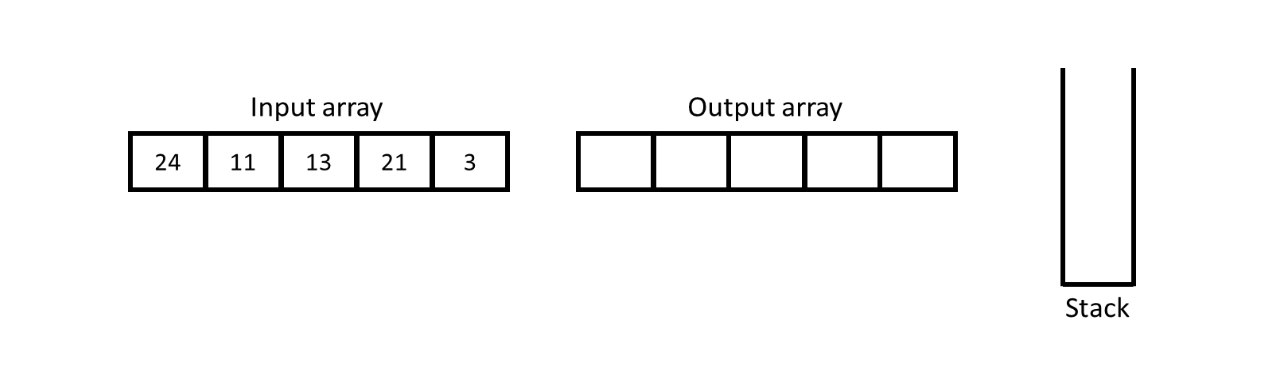Initially, input array will be given and an output array with stack will be created.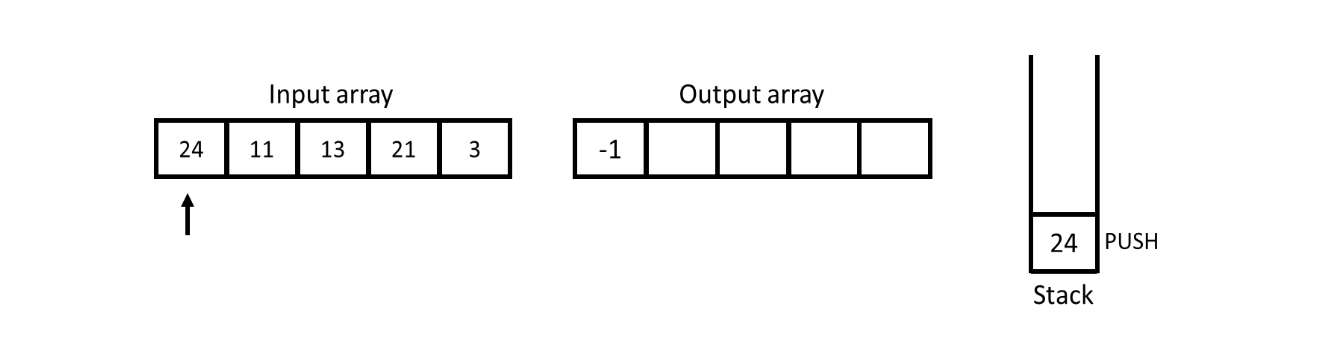Start traversing of array from the left side and for the leftmost element nearest greater to left will be `-1` and put the value from the input array into the stack for further comparision.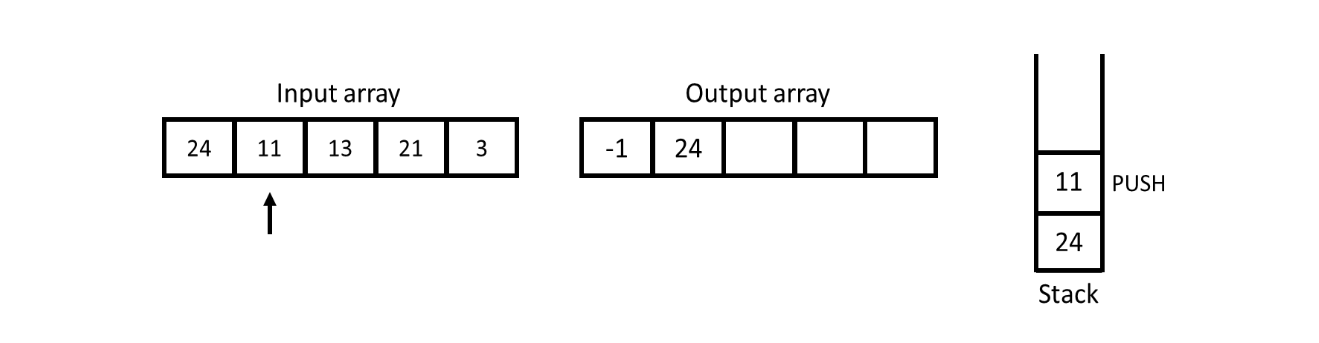For 11, stack is not empty so we have to check the top most value if it is greater than 11 or not. Here we observe that 11 is smaller than 24 so 24 will be inserted into output array and 11 will be pushed into the stack.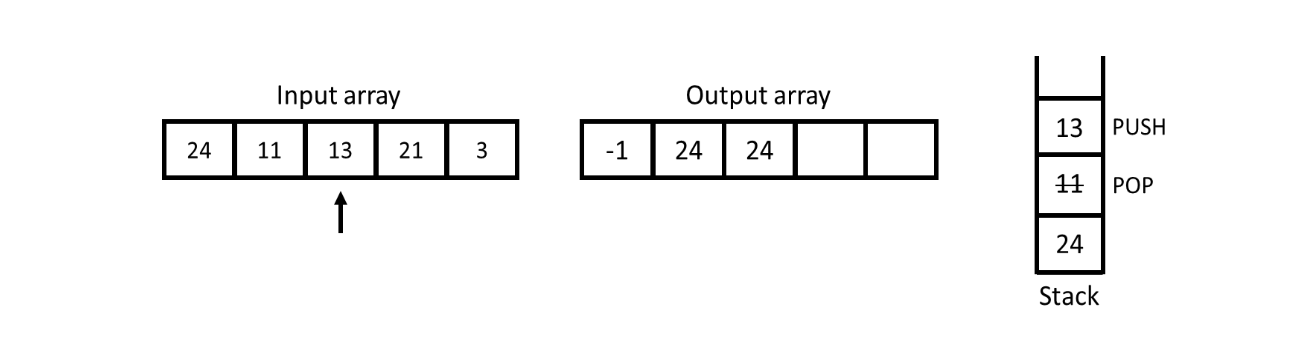For 13, stack is not empty so check the top of stack and the top of stack i.e., 11 is smaller than 13 so pop off 11 from the stack again check the top of stack. Now the top of stack is 24 and it is greater than than 13 so insert 24 into the output array and push 13 into the stack.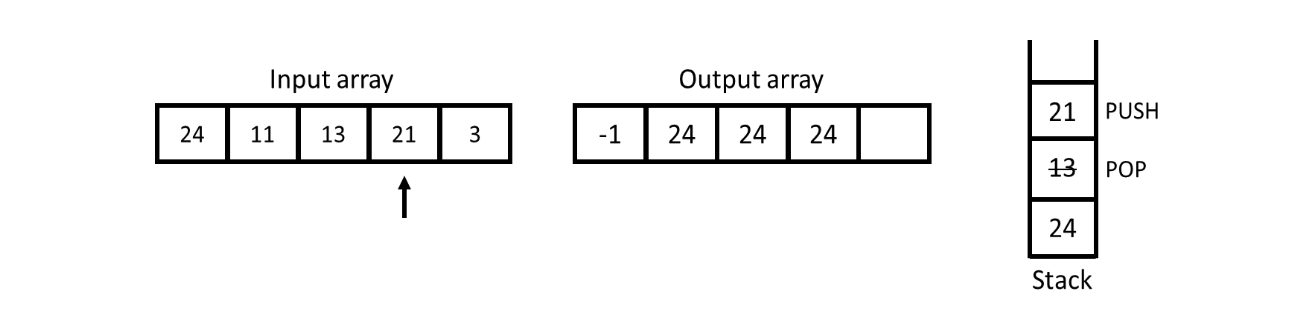For 21, the top of stack is 13 which is smaller than 21 so pop off 13 and again check the top of stack. Now, top of stack is 24 which is greater than 21 so insert 24 into the output array and push 21 into the stack.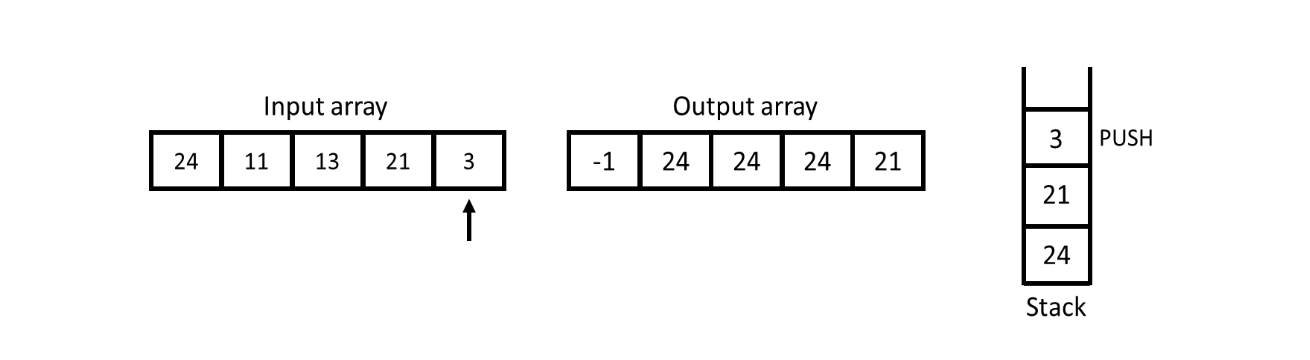For 3, the top of stack is 21 which is obviously greater than 3 so insert 21 into the output array and push 3 into the stack.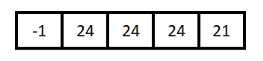At the this point all the elements are covered and iterations are complete. Now, return the output array.

### Program

Python
``````class Stack:
def __init__(self):
self.stack = []

def is_empty(self):
return len(self.stack) == 0

def push(self, num):
self.stack.append(num)

def pop(self):
if self.is_empty():
raise Exception('Stack Underflow')
return self.stack.pop()

def peek(self):
if self.is_empty():
return None
return self.stack[-1]

def nearest_greater_to_left(arr):
stack = Stack()
result = []

for i in range(0, len(arr)):
if stack.is_empty():
result.append(-1)
stack.push(arr[i])
elif not stack.is_empty():
while(not stack.is_empty() and arr[i] > stack.peek()):
stack.pop()
if stack.is_empty():
result.append(-1)
else:
result.append(stack.peek())
stack.push(arr[i])

return result

arr = [1, 6, 4, 10, 2, 5]
print(nearest_greater_to_left(arr))
``````
JavaScript
``````class Stack {
constructor() {
this.stack = [];
}

isEmpty() {
return this.stack.length === 0;
}

push(num) {
this.stack.push(num);
}

pop() {
if (this.isEmpty()) {
throw "Stack Underflow";
}
return this.stack.pop();
}

peek() {
if (this.isEmpty()) {
return null;
}
return this.stack[this.stack.length - 1];
}
}

function nearestGreaterToLeft(arr) {
const stack = new Stack();
result = [];

for (let i = 0; i < arr.length; i++) {
if (stack.isEmpty()) {
result.push(-1);
stack.push(arr[i]);
} else if (!stack.isEmpty()) {
while (!stack.isEmpty() && arr[i] > stack.peek()) {
stack.pop();
}
if (stack.isEmpty()) {
result.push(-1);
} else {
result.push(stack.peek());
}
stack.push(arr[i]);
}
}

return result;
}

arr = [1, 6, 4, 10, 2, 5];
console.log(nearestGreaterToLeft(arr));
``````

Output

``````[-1, -1, 6, -1, 10, 10]
``````